# Parallelogram Law of Vector Resolution

According to the parallelogram law of vector addition:

“If two vector quantities are represented by two adjacent sides or a parallelogram then the diagonal of parallelogram will be equal to the resultant of these two vectors.”

Consider two vectors V1  & V2. Let the vectors have the following orientation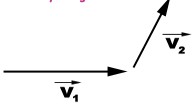parallelogram of these vectors is :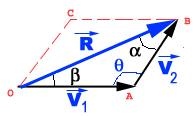According to parallelogram law: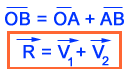The process of determining the magnitude of a vector is known as vector resolution.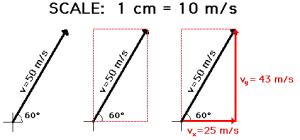Magintude or resultant vector can be determined by using either sine law or cosine law.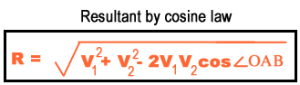### Feedback is important to us.#### Satya Prakash

Mr. Satya Prakash is a research scholar.

### One thought on “Parallelogram Law of Vector Resolution”

•21/09/2015 at 11:15 PM